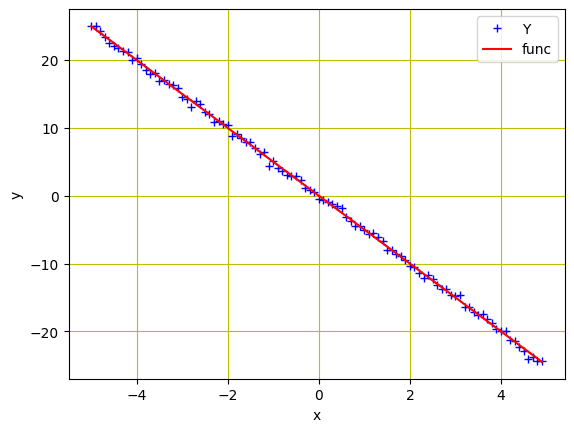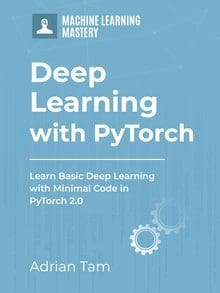# Implementing Gradient Descent in PyTorch

Last Updated on April 8, 2023

The gradient descent algorithm is one of the most popular techniques for training deep neural networks. It has many applications in fields such as computer vision, speech recognition, and natural language processing. While the idea of gradient descent has been around for decades, it’s only recently that it’s been applied to applications related to deep learning.

Gradient descent is an iterative optimization method used to find the minimum of an objective function by updating values iteratively on each step. With each iteration, it takes small steps towards the desired direction until convergence, or a stop criterion is met.

In this tutorial, you will train a simple linear regression model with two trainable parameters and explore how gradient descent works and how to implement it in PyTorch. Particularly, you’ll learn about:

• Gradient Descent algorithm and its implementation in PyTorch
• Batch Gradient Descent and its implementation in PyTorch
• Stochastic Gradient Descent and its implementation in PyTorch
• How Batch Gradient Descent and Stochastic Gradient Descent are different from each other
• How loss decreases in Batch Gradient Descent and Stochastic Gradient Descent during training

Kick-start your project with my book Deep Learning with PyTorch. It provides self-study tutorials with working code.

So, let’s get started.Picture by Michael Behrens. Some rights reserved.

## Overview

This tutorial is in four parts; they are

• Preparing Data
• Plotting Graphs for Comparison

## Preparing Data

To keep the model simple for illustration, we will use the linear regression problem as in the last tutorial. The data is synthetic and generated as follows:

Same as in the previous tutorial, we initialized a variable X with values ranging from $-5$ to $5$, and created a linear function with a slope of $-5$. Then, Gaussian noise is added to create the variable Y.

We can plot the data using matplotlib to visualize the pattern:Data points for regression model

### Want to Get Started With Deep Learning with PyTorch?

Take my free email crash course now (with sample code).

Click to sign-up and also get a free PDF Ebook version of the course.

Now that we have created the data for our model, next we’ll build a forward function based on a simple linear regression equation. We’ll train the model for two parameters ($w$ and $b$). We will also need a loss criterion function. Because it is a regression problem on continuous values, MSE loss is appropriate.

Before we train our model, let’s learn about the batch gradient descent. In batch gradient descent, all the samples in the training data are considered in a single step. The parameters are updated by taking the mean gradient of all the training examples. In other words, there is only one step of gradient descent in one epoch.

While Batch Gradient Descent is the best choice for smooth error manifolds, it’s relatively slow and computationally complex, especially if you have a larger dataset for training.

### Training with Batch Gradient Descent

Let’s randomly initialize the trainable parameters $w$ and $b$, and define some training parameters such as learning rate or step size, an empty list to store the loss, and number of epochs for training.

We’ll train our model for 20 epochs using below lines of code. Here, the forward() function generates the prediction while the criterion() function measures the loss to store it in loss variable. The backward() method performs the gradient computations and the updated parameters are stored in w.data and b.data.

Here is the how the output looks like and the parameters are updated after every epoch when we apply batch gradient descent.

Putting all together, the following is the complete code

The for-loop above prints one line per epoch, such as the following:

As we learned that batch gradient descent is not a suitable choice when it comes to a huge training data. However, deep learning algorithms are data hungry and often require large quantity of data for training. For instance, a dataset with millions of training examples would require the model to compute the gradient for all data in a single step, if we are using batch gradient descent.

This doesn’t seem to be an efficient way and the alternative is stochastic gradient descent (SGD). Stochastic gradient descent considers only a single sample from the training data at a time, computes the gradient to take a step, and update the weights. Therefore, if we have $N$ samples in the training data, there will be $N$ steps in each epoch.

### Training with Stochastic Gradient Descent

To train our model with stochastic gradient descent, we’ll randomly initialize the trainable parameters $w$ and $b$ as we did for the batch gradient descent above. Here we’ll define an empty list to store the loss for stochastic gradient descent and train the model for 20 epochs. The following is the complete code modified from the previous example:

This prints a long list of values as follows

## Plotting Graphs for Comparison

Now that we have trained our model using batch gradient descent and stochastic gradient descent, let’s visualize how the loss decreases for both the methods during model training. So, the graph for batch gradient descent looks like this.The loss history of batch gradient descent

Similarly, here is how the graph for stochastic gradient descent looks like.Loss history of stochastic gradient descent

As you can see, the loss smoothly decreases for batch gradient descent. On the other hand, you’ll observe fluctuations in the graph for stochastic gradient descent. As mentioned earlier, the reason is quite simple. In batch gradient descent, the loss is updated after all the training samples are processed while the stochastic gradient descent updates the loss after every training sample in the training data.

Putting everything together, below is the complete code:

## Summary

In this tutorial you learned about the Gradient Descent, some of its variations, and how to implement them in PyTorch. Particularly, you learned about:

• Gradient Descent algorithm and its implementation in PyTorch
• Batch Gradient Descent and its implementation in PyTorch
• Stochastic Gradient Descent and its implementation in PyTorch
• How Batch Gradient Descent and Stochastic Gradient Descent are different from each other
• How loss decreases in Batch Gradient Descent and Stochastic Gradient Descent during training

## Get Started on Deep Learning with PyTorch!#### Learn how to build deep learning models

...using the newly released PyTorch 2.0 library

Discover how in my new Ebook:
Deep Learning with PyTorch

It provides self-study tutorials with hundreds of working code to turn you from a novice to expert. It equips you with
tensor operation, training, evaluation, hyperparameter optimization, and much more...

### 2 Responses to Implementing Gradient Descent in PyTorch

1.Evgeny April 30, 2023 at 3:56 am #

Thank you for this code, it was quite helpful in my study. Unfortunately, SGD does not work.
But I suggest couple of corrections:
1) Just to beautify in 10th line: Y = func + torch.randn(X.size())
2) To make SGD works (here it is not convergent): step_size=0.001 for SGD only
3) To make SGD fits it description in wiki, make data shuffle before each epoch: